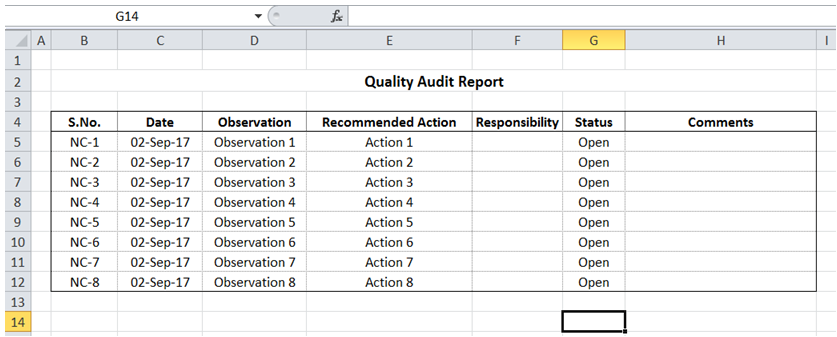Vba shade alternative rows in Excel

For example, in the table below, I would like to shade alternate rows.To do it in Excel, here is the answer:

1. Option Explicit
3. Dim i As Integer
4. ''
5. For i = 5 To 12
6. If i Mod 2 = 0 Then
7. ActiveSheet.Range(ActiveSheet.Cells(i, 2), ActiveSheet.Cells(i, 8)).Select
8. With Selection.Interior
9. .Pattern = xlSolid
10. .PatternColorIndex = xlAutomatic
11. .ThemeColor = xlThemeColorAccent6
14. End With
15. End If
16. Next i
17. End Sub

Description:

a) The code loops through the table range. Line 6 "i Mod 2 =0" is the criteria to apply formatting - basically even numbered rows have shading. When this criteria is met, the cell range is shaded.